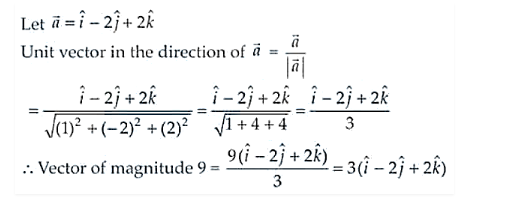# The vector in the direction of the vector

Question:

The vector in the direction of the vector $\hat{i}-2 \hat{j}+2 \hat{k}$ that has magnitude 9 is

(A) $\hat{i}-2 \hat{j}+2 \hat{k}$

(B) $\frac{\hat{i}-2 \hat{j}+2 \hat{k}}{3}$

(C) $3(\hat{i}-2 \hat{j}+2 \hat{k})$

(D) $9(\hat{i}-2 \hat{j}+2 \hat{k})$

Solution:

The correct option is (C).